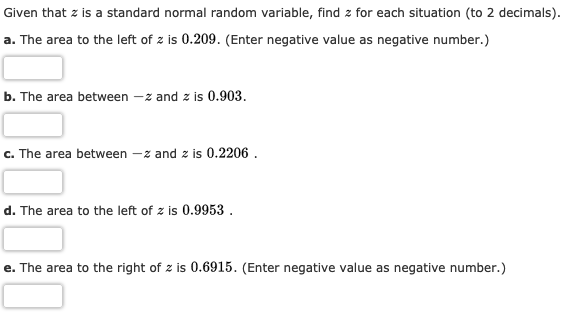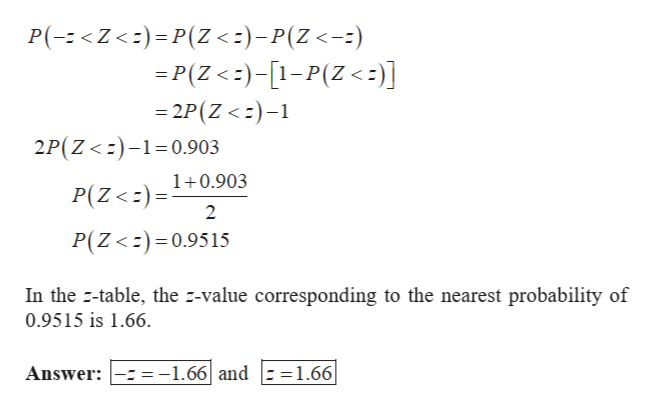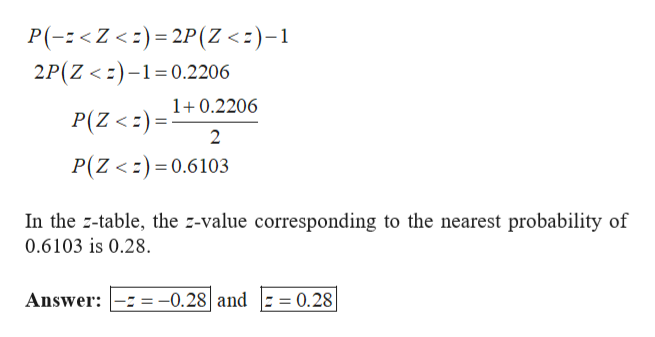Given that z is a standard normal random variable, find z for each situation (to 2 decimals)a. The area to the left of z is 0.209. (Enter negative value as negative number.)b. The area between -z and z is 0.903-z and z is 0.2206c. The area betweend. The area to the left of z is 0.9953 .e. The area to the right of z is 0.6915. (Enter negative value as negative number.)

Questionhelp_outlineImage TranscriptioncloseGiven that z is a standard normal random variable, find z for each situation (to 2 decimals) a. The area to the left of z is 0.209. (Enter negative value as negative number.) b. The area between -z and z is 0.903 -z and z is 0.2206 c. The area between d. The area to the left of z is 0.9953 . e. The area to the right of z is 0.6915. (Enter negative value as negative number.) fullscreen
Step 1

a.

The area to the left of z is 0.209 can be written as P(Z < z)=0.209.

In the z-table for negative values of z, the z-value corresponding to the nearest probability of 0.209 is -0.81.

b.

The area between –z and z is 0.903 can be written as P(-z <Z <z) = 0.903.help_outlineImage TranscriptioncloseP(-Z<)P(Z <-)-P(Z<--) P(Z<)-1-P(Z<:)] -2P (Z <)- 2P(Z<)-1 0.903 1+0.903 P(Z
Step 2

c.

The area between –z and z is 0.2206 can be written as P(-...help_outlineImage TranscriptioncloseP(-Z <)2P(Z <)-1 2P(Z <)1 0.2206 1+0.2206 P(Z

Want to see the full answer?

See Solution

Want to see this answer and more?

Our solutions are written by experts, many with advanced degrees, and available 24/7

See Solution
Tagged in

Other